# Refraction

## Refraction

The following text is used only for teaching, research, scholarship, educational use and informative purpose following the fair use principles.

We thank the authors of the texts and the source web site that give us the opportunity to share their knowledge

# Refraction

Refraction is the bending of a wave as it passes from one medium to another*.

When light travels from a rarer to a denser medium it is refracted towards the normal and vice versa.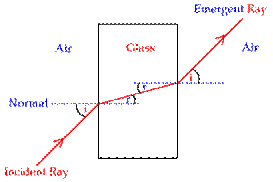The two Laws of Refraction of Light

• The incident ray, the normal and the refracted ray all lie on the same plane.

(not to be confused with the reflected ray, from the laws of reflection of light).

• The ratio of the sin of the sin of the angle of incidence to the sin of the angle of refraction is a constant called the Refractive Index.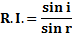The refractive index between any two media is a constant and is given the symbol ŋ.
The second law of refraction is also known as Snell’s Law*.

This leads to the following definition:
The Refractive Index of a Medium
is the ratio of the sine of the angle of incidence to the sine of the angle of refraction when light travels from a vacuum into that medium*.

Note that if you see the phrase “the Refractive Index of glass is 1.5”, it means that when light travels from air into glass the refractive Index is 1.5*.
This is written as wŊg = 1.5
This is important because is light is going from glass into air there is a different refractive index.

Real Depth and Apparent Depth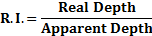See problems 5 and 6, page 34, and try questions 1 – 5 page 34.
Question 5 is challenging but worth a go – draw a diagram.

Refractive Index in Terms of Relative Speeds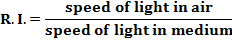or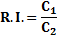Where C1 is the speed of light in the first medium and C2 is the speed of light in the second medium*.
Usually one of these media will be air.
Now look at problems 7 and 8, then try questions 1–4, page 36.

Total Internal Reflection

When the angle of incidence in the denser of two media reaches a certain (critical) angle, the light no longer refracts outward, but instead travels along the surface (see diagram).

Total internal reflection occurs when the angle of incidence in the denser of the two media is greater than the critical angle and light is reflected back into the denser medium.

The angle of refraction is now 900, and this leads to a definition of the critical angle.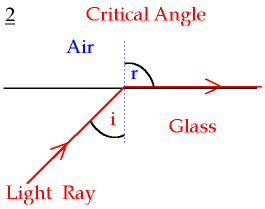The critical angle corresponds to the angle of incidence in the denser of two media which causes the angle of refraction to be 900.

Relationship between Critical Angle and Refractive Index*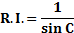Where c represents the critical angle.
Remember Refractive Index of a medium is defined in relation to light going from air into that medium.
Now look over examples 9 and 10, page 38. Then try questions 1 – 6, page 38

Using a prism to turn a ray of light through (i) 900 (ii) 1800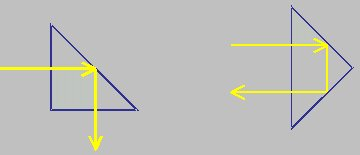Applications of Total Internal Reflection

• Optical Fibres
• Endoscopes
• Periscopes

Optical Fibres*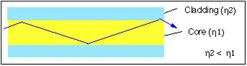• An optical fibre consists of a glass pipe coated with a second material of lower refractive index.
• Light enters one end of the fibre and strikes the boundary between the two materials at an angle greater than the critical angle, resulting in total internal reflection at the interface.
• This reflected light now strikes the interface on the opposite wall and gets totally reflected again.
• This process continues all along the glass pipe until the light emerges at the far end.

Note:

• If the second cladding material wasn’t there or had a refractive index greater than that of the central core total internal reflection would not occur and the light would simply escape out.
• The outer cladding also acts as a protective layer against scratches etc.

Applications

• Telecommunications
• Medicine (endoscopes)

Advantages of optical fibres over copper conductors
Less interference / boosted less often / cheaper raw material / occupy less space / more information (carried) in the same space / flexible for inaccessible places/ do not corrode

Summary of FormulaMandatory Experiment:
To verify Snell’s Law and use it to measure the refractive index of a solid.

Leaving Cert Physics Syllabus: Refraction

 Content Depth of Treatment Activities STS 1. Laws of refraction Refractive index   Refractive index in terms of relative speeds. Demonstration using ray box or laser or other suitable method. Appropriate calculations. Appropriate calculations. Practical examples, e.g. real and apparent depth of fish in water. 2. Total internal reflection Critical angle Relationship between critical angle and refractive index. Transmission of light through optical fibres. Demonstration. Appropriate calculations Reflective road signs. Mirages. Prism reflectors. Uses of optical fibres: telecommunications medicine (endoscopes) Experiments: Verification of Snell’s law of refraction. Measurement of the refractive index of a liquid or a solid.

VERIFICATION OF SNELL’S LAW OF REFRACTION
Or
TO MEASURE THE REFRACTIVE INDEX OF A GLASS BLOCK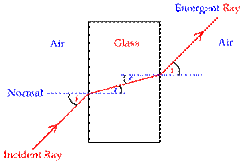APPARATUS: Glass block, ray-box, protractor, page

DIAGRAM (I couldn’t find one which included the ray-box)

PROCEDURE

• Place a glass block on the page and mark its outline.
• Shine a ray of light from the ray-box into the glass block.

• Mark two dots on the incident ray and exit ray and draw the outline of the block.
• Remove the block and complete all lines including the normal, as indicated on the diagram.

• Measure the angle of incidence i and angle of refraction r using the protractor.
• Repeat for different values of i.

• Draw up a table as shown.
• Plot a graph of sin i against sin r. A straight line through the origin verifies Snell’s law of refraction

i.e. sin i ∝ sin r.

• The slope of the line gives a value for the refractive index of glass.

• The refractive index of glass is also equal to the average value of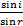RESULTS

 i (degrees) r (degrees sin i sin r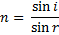Average value for refractive index =

CONCLUSION
The refractive index based on the slope of the graph was 1.47, and from the table of data we got an average of 1.44 so all in all, not a bad day’s work.

SOURCES OF ERROR / PRECAUTIONS

• Using small angles of incidence will result in large percentage errors.
• Place two dots far apart on the incident and refracted light beams to accurately locate the beams

Extra Credit
*Refraction is the bending of a wave as it passes from one medium to another.
Its etymological derivation comes from the past participle of the lati verb 'to break'.  This suggests, by analogy, a broken bone whose alignment changes abruptly at the place of fracture.

*Snell’s Law
Utterly useless trivia #1: The law of refraction was first accurately described by Ibn Sahl, of Baghdad, in 984.
Utterly useless trivia #2: In French, Snell's Law is called "la loi de Descartes" or "loi de Snell-Descartes" because the French philosopher/mathematician/scientist came up with the law independently in 1637 (Snell devised his version in 1621).

Ah, but here is the very much non useless trivia bit: how does the light know how much to bend when it hits the water/air interface (let’s assume it’s going from air to water)? This is a very disconcerting issue – it seems that light follows the path of least time – i.e. it takes the quickest route from A to B, which is obviously a straight line in a given medium, but when it’s passing from one medium to another it bends in such a way that its overall journey-time is a minimum. The physicist Richard Feynman gave the following scenario to explain the concept: a lifeguard on a beach spots a swimmer in trouble some distance away, in a diagonal direction. He can run three times faster than he can swim. What is the quickest path to the swimmer?
But I ask again – how does light know how much to bend by?

*When light travels from a vacuum into that medium
In practice we consider air and a vacuum to be equivalent.

*In other words if you see the phrase “the Refractive Index of glass is 1.5”, it means that when light travels from air into glass the refractive Index is 1.5
This is important to remember because if light is going in the other direction (i.e. from glass to air, the refractive index will not be 1.5.
This often causes confusion, especially when we go on to define the critical angle.
*Ŋ = c1/c2
Why is "c" used for the speed of light?
From the Latin 'celeritas', meaning speed, as an alternative to v (for particles).

*Relationship between Critical Angle and Refractive Index
The derivation of this formula is on pages 37/38 – you don’t need to know it, but it might be useful to see if you can follow it.

*Optical Fibres
Irishman John Tyndall - from County Carlow of all places (who would have guessed?) was one of first to investigate fibre optics, and used to demonstrate it using a water fountain.
Tyndall was one of the foremost scientists in Britain in the mid to late 1800’s and did a lot of pioneering work on optics. He was the first to explain properly why the sky is blue and why it gets red as the sun goes down. He was also a noted mountaineer.
Never again ask why God invented Carlow.
BTW:
Japan has successfully test an optic fibre which can take14 trillion bits per second down a single strand. That’s equivalent to over 2,500 CDs every second. This capacity is tripling every 6 months and is expected to continue doing so for the next 20 years. Source: Shift Happens (YouTube)

Experiments
There are three mandatory experiments on the syllabus to do with refraction
To verify Snell’s Law
To measure the refractive index of a liquid or a solid.
The or in italics implies that you must be given the option, and therefore the exam question cannot specify measuring the refractive index of a liquid.
But in verifying Snell’s Law, we plot a graph of Sin i against Sin r, and show that because the graph is a straight line going through the origin, the two variables are directly proportional.
To calculate the refractive index we then simply calculate the slope of the graph, and if Sin i is on the y-axis, the slope corresponds to the refractive index.
No need to worry about measuring the refractive index of any messy liquids!
Hints for carrying out the experiment:
Start with a large angle for incidence and measure the corresponding angle of refraction.
Now reduce this angle at roughly regular intervals each time to ensure a wide range of values.

Exam questions:  Refraction

• [2005 OL]
• What is meant by the reflection of light?
• State the laws of reflection of light.
• Describe an experiment to demonstrate one of the laws of reflection of light.
• [2010 OL]

Which of these scientists is associated with the law of refraction of light?
Rutherford Snell Joule Einstein

• [2002 OL][2004 OL][2005 OL][2009 OL]

What is meant by refraction of light?

• 

State Snell’s law of refraction.

• [2002 OL]

State the laws of refraction of light.

• [2004 OL]

What is meant by the refractive index of a material?

• 
• Light is refracted as it enters the cornea from air as shown in the diagram.

Calculate the refractive index of the cornea.

• Draw a diagram to show the path of a ray of light as it passes from water of refractive index 1.33 into the cornea.
• [2005 OL]
• What special name is given to the angle of incidence i, when the effect shown in the diagram occurs?
• In the diagram the value of the angle i is 41.80. Calculate a value for the refractive index of the glass.
• Draw a diagram to show what happens to the ray of light when the angle of incidence i is increased to 450.

• [2003OL]

Explain, with the aid of a labelled diagram (i) critical angle, (ii) total internal reflection

• [2004 OL]

When will total internal reflection occur?

• [2004 OL]

Define the critical angle.

• 

The refractive index of a liquid is 1.35, what is the critical angle of the liquid?

• [2003 OL]

The critical angle for the glass is 42o. Calculate the refractive index of the glass.

• 

Calculate the critical angle for diamond. The refractive index of diamond is 2.4.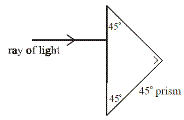• 

What is the critical angle of a sample of glass whose refractive index is 1.46?

• [2003 OL]
• The diagram shows a 45o prism made of glass and a ray of light entering the prism from air. Copy the diagram and show the path of the ray through the prism and back into the air.
• Explain why the ray follows the path that you have shown.
• [2003 OL][2005 OL]

Give two uses of total internal reflection.

Optical fibres

• [2009 OL]

Draw a diagram to show the path of a ray of light travelling through an optical fibre.

• [2004 OL]

Explain how a signal is transmitted along an optical fibre.

• [2004 OL]

How is the escape of light from the sides of an optical fibre prevented?

• 

An optical fibre has an outer less dense layer of glass. What is the role of this layer of glass?

• [2004 OL]

Give one use for optical fibres.

• 

Give two reasons why the telecommunications industry uses optical fibres instead of copper conductors to transmit signals.

• 

Impurities in glass reduce the power transmitted in an optic fibre by half every 2 km.
The initial power being transmitted by the light is 10 W.
What is the power being transmitted by the light after it has travelled 8 km through the fibre?

• 

Information is transmitted over long distances using optical fibres with a refractive index of 1.55.
What is the speed of the light as it passes through the fibre?

• 

An optical fibre is manufactured using glass of refractive index of 1.5.
Calculate the speed of light travelling through the optical fibre.

• [2004 OL]

Name a material that is used in the manufacture of optical fibres.

Mandatory Experiments

• [2008 OL]

An experiment was carried out to measure the refractive index of a substance.
The experiment was repeated a number of times.

• Draw a labelled diagram of the apparatus that could be used in this experiment.
• What measurements were taken during the experiment?
• How was the refractive index of the substance calculated?
• Why was the experiment repeated?

• [2006 OL]

A student carried out an experiment to verify Snell’s law of refraction by measuring the angle of incidence i and the angle of refraction r for a ray of light entering a glass block. The student repeated this procedure two more times. The data recorded by the student is shown in the table.

 angle of incidence i angle of refraction r sin i sin i sin i/sin r 30o 19o 45o 28o 65o 37o

• Draw a labelled diagram of the apparatus used in the experiment.
• Describe how the student found the position of the refracted ray.
• How did the student measure the angle of refraction?
• Use the data to verify Snell’s law of refraction.

• 
 i / ° 30 40 50 55 60 65 70 r / ° 19 26 30 33 36 38 40

In an experiment to verify Snell’s law, a student recorded the following data.

• Draw a labelled diagram of the apparatus used.

On your diagram, indicate an angle i and its corresponding angle r.

• Using the recorded data, draw a suitable graph
• Explain how your graph verifies Snell’s law.
• Using your graph, find the refractive index
• The student did not record any values of i below 30°, give two reasons why?

• 
 i/degrees 20 30 40 50 60 70 r/degrees 14 19 26 30 36 40

In an experiment to verify Snell’s law, a student measured the angle of incidence i and the angle of refraction r for a ray of light entering a substance. This was repeated for different values of the angle of incidence. The following data was recorded.

• Describe, with the aid of a diagram, how the student obtained the angle of refraction.
• Draw a suitable graph on graph paper and explain how your graph verifies Snell’s law.
• From your graph, calculate the refractive index of the substance.
• The smallest angle of incidence chosen was 200.

Why would smaller values lead to a less accurate result?

Exam solutions:  Refraction

•
• Reflection is the bouncing of light from a surface.
• The incident ray, the normal at the point of incidence and the reflected ray all lie on the same plane.

The angle of incidence is equal to the angle of reflection (i = r).

• Apparatus: raybox, mirror.

Procedure: mark the position of the rays and measure the angles i and r.
Observation/conclusion: i = r.

• Snell
• Refraction of light is the bending of light as it passes from one medium to another (of different refractive index).
• The ratio of the sin of the sin of the angle of incidence to the sin of the angle of refraction is a constant.
• The incident ray, the normal and the refracted ray all lie on the same plane.

The ratio of the sin of the sin of the angle of incidence to the sin of the angle of refraction is a constant.

• n = sin i/ sin r,  where i is the angle of incidence and r is the angle of refraction.
•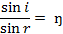•

Þ n = sin 37/sin 27          Þ  n = 1.33

• Both media have the same refractive index so there is no bending of light so draw a straight line passing from one medium to the other without bending.
•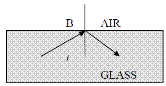• It is called the critical angle.
• n= 1/ sin C            Þ        n = 1/sin 41.80              Þ n = 1/0.67 Þ n = 1.5.

• See diagram.

•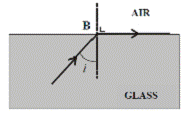• The critical angle corresponds to the angle of incidence in the denser of two media which causes the angle of refraction to be 900.
• Total internal reflection occurs when the angle of incidence in the denser of the two media is greater than the critical angle and light is reflected back into the denser medium.
• When the angle of incidence is greater than the critical angle.

• The critical angle corresponds to the angle of incidence in the denser of two media which causes the angle of refraction to be 900.
• ng =1/ sin c            Þ c = 47.80
• n = 1/sin C            Þ n = 1/ sin 42                       Þ n = 1.5
• n = 1/ sin c Þ c = 24.6 0.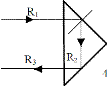•  n = 1/sin c            Þ c = 43.2o
•

• See diagram
• Because total internal reflection occurs twice while inside the prism.
• Fibre optics, endoscopes, reflective road signs, telecommunications, binoculars, periscope.

Optical fibres

•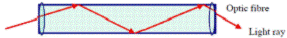Answer:

•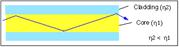An optical fibre consists of a glass pipe coated with a second material of lower refractive index.
• Light enters one end of the fibre and strikes the boundary between the two materials at an angle greater than the critical angle, resulting in total internal reflection at the interface.
• This reflected light now strikes the interface on the opposite wall and gets totally reflected again.
• This process continues all along the glass pipe until the light emerges at the far end.

• Total internal reflection occurs due to an outer cladding of lower refractive index.
• Total internal reflection will only occur if the outer medium is of lesser density (strictly speaking it should read ‘lower refractive index’ rather than ‘less dense’, but there you go.)

It also prevents damage to the surface of the core.

• Endoscope, telecommunications, binoculars.
• Less interference, boosted less often, cheaper raw material, occupy less space,  more information carried in the same space, flexible for inaccessible places, do not corrode, etc.
• After 2 km power has dropped to 5 W; after 4 km power has dropped to 2.5 W; after 6 km power has dropped to 1.25 W; after 8 km power has dropped to 0.625 W.
• n = cair/cglass           Þ cglass = 3.0 × 108/1.55                     Þ        cglass = 1.94 × 108 m s-1
• ng =  ca /cg  Þ        1.5 = 3 × 108/ vg          Þ        vg = 2.0 × 108 m s-1
• Glass / plastic / sand / silicon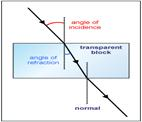Mandatory Experiments

•
• As in diagram, plus a ray box and protractor.
• The angle of incidence and the angle of refraction.
• By using the formula n = sin i ÷ sin r.
• To increase the accuracy of the results.
•
• See diagram. Also include a protractor and raybox.
•Draw the incident ray going in, the emergent ray coming out, then remove the block and join the two lines. This represents the refracted ray.
• By measuring the angle between the normal and the refracted ray using a protractor.

 angle of incidence i angle of refraction r sin i sin i sin i/sin r 30o 19o 0.500 0.326 1.53 45o 28o 0.707 0.469 1.51 65o 37o 0.906 0.602 1.50
•

• In each case sin i/sin r is (approximately) constant; therefore this verifies Snell’s Law.

•
• Diagram to show:

A target medium e.g. glass block
Incident ray (from ray box)
Perpendicular / normal and refracted ray
Label angles i and r

• Correct sin i and sin r values for six points
 sin i 0.5 0.643 0.766 0.819 0.866 0.906 0.939 sin r 0.325 0.438 0.5 0.544 0.588 0.615 0.643

Label axes correctly on graph paper
Plot six points correctly
Straight line showing good distribution

• A straight line through the origin shows that sin i is proportional to sin r
• Correct slope method

(n = ) 1.41 [range: 1.38 – 1.52]

• To reduce the (percentage) error

Elaboration e.g. difficult to measure /read angles, r < i , etc.

•
• See diagram, plus ray-box.

Mark the position of the incident and exit rays and also the outline of the block.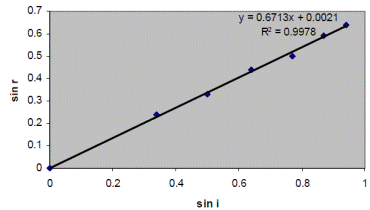Remove the block then measure the angle between the refracted ray and the normal using a protractor.

 sin i 0.34 0.5 0.64 0.77 0.87 0.94 sin r 0.24 0.33 0.44 0.5 0.59 0.64
•
• Refractive index = slope  =  y2 – y1 / x2 – x1         Þ n = 1.49
• There would be a greater percentage error associated with measuring smaller angles.

Source : http://www.thephysicsteacher.ie/LC%20Physics/Student%20Notes/4.%20Refraction.doc

Author : not indicated on the source document of the above text

If you are the author of the text above and you not agree to share your knowledge for teaching, research, scholarship (for fair use as indicated in the United States copyrigh low) please send us an e-mail and we will remove your text quickly.

### Refraction

#### Refraction

Main page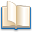Alimentation - nutritionAnimalsArchitectureArtAstrology, esoteric, parapsychology and fantasyAstronomyBiology and geneticsBotany and agricultureChemistryCourses, training, guides, handbooks and tipsCulture General and actualityDermatology, health and wellnessDictionaries and glossariesEconomics and financeEducation and pediatricsEngineering and technologyEnglish grammar and anthologyFashion and show businessFitnessFood, recipes and drinkGames, play spaceGeography, geology and environmentHistoryInformatics and computer worldJobs worldLanguagesLeisure and DIY do it yourselfLessons for studentsLiterature, biographies and journalismMathematics, geometry and statisticalMedicineMusicNotes and concept mapsPhilosophyPhotography and images - picturesPhysicsPhysiologyPsychology and psychoanalysisQuiz questions and answersReligionsRights law and political scienceScienceSociology and cultural anthropologySportSummaries and reviewsTelevision, film - movies and comicsTheater and communicationsTheses, themes and dissertationsTravel and tourist guidesUseful documents and tablesUtility, calculators and convertersWeather and meteorology

#### Refraction

This is the right place where find the answers to your questions like :

Who ? What ? When ? Where ? Why ? Which ? How ? What does Refraction mean ? Which is the meaning of Refraction?

##### Refraction physics notes

Alanpedia.com from 1998 year by year new sites and innovations